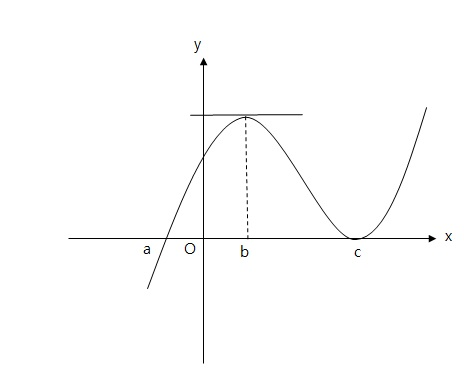Calculus

# Increasing / Decreasing Functions

What is the range of all possible values $a$ such that $f(x) = x^3-ax^2+(a+6)x+1$ is an everywhere increasing function?

What is the range of possible values $a$ such that the function $f(x) = -x^3+ax^2-18x-1$ is an always decreasing function?The above diagram shows the curve $y=f(x).$ Let $a$ be the $x$-coordinate of the intersection point between the curve and negative part of the $x$-axis, and let $b$ and $c$ be the $x$-coordinates of the local maximum and minimum of the curve, respectively, as shown in the diagram. If $a = -3, b = 4, c = 7,$ and $f'(x)>0$ for the portions of the curve that are not displayed in the diagram, what is the range of $x$ that satisfies $f(x)f'(x) > 0?$

What is the range of possible values of $a$ such that the function $f(x)=3x^4 - 4(a+7)x^3+6(7a+6)x^2-72ax+1$ increases in the interval $x \geq 6?$

What is the sum of the minimum and maximum values of the constant $a$ such that $f(x) = \frac{1}{3}x^3 + ax^2 + (14 a - 40)x + 12$ is an increasing function in the interval $(-\infty,\ \infty)?$

×